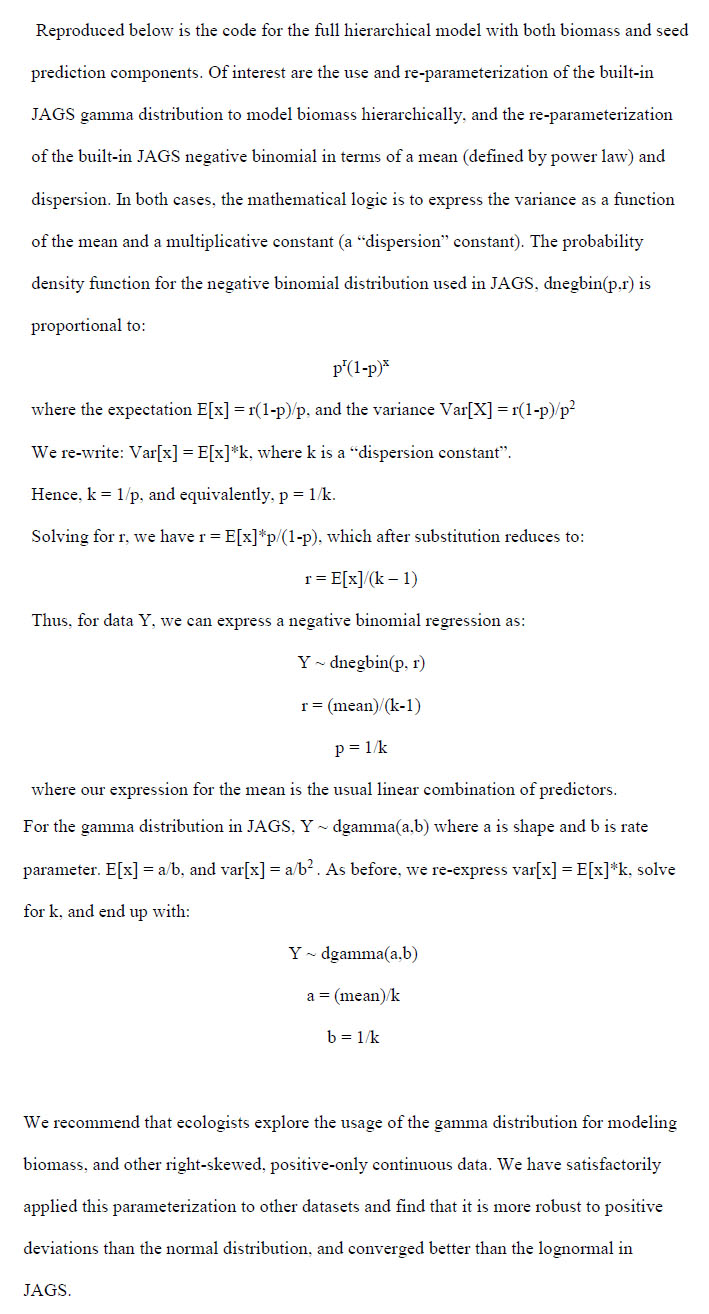Ecological Archives E096-096-A5

Chris H. Wilson, T. Trevor Caughlin, David J. Civitello, and S. Luke Flory. 2015. Combining mesocosm and filed experiments to predict invasive plant performance: a hierarchical Bayesian approach. Ecology 96:10841092. http://dx.doi.org/10.1890/14-0797.1

Appendix E. Details on model development, particularly working with the gamma and negative binomial parameterizations in JAGS, plus pseudo-code for generating cross-validated posterior predictions.Pseudo-code for running cross-validation checks:

```

sink("ranmodelgroE.txt") #the sink function drops the code as a text file into your working directory
cat('

# Repeat model specification from above

}

',fill=TRUE)
sink()

# data block: define your data inputs here

# The following code sets observations in the dataset to NA
# in our case, this is based on the held-out site

um<-unique(massdata\$sites)
loocv<-vector("list",length(um))

for(i in 1:length(um)) {

mdat<-massdata
mdat\$totmass[which(mdat\$Site==um[i])]<-NA
loocv[[i]]<-mdat
}

# This code block creates a list-vector to save the results of the  #multiple model fits in
# and then  executes a loop to fit the model N times, where N= number #of hold-out sites, or
# whatever

results<-vector("list",length(um))
set.seed(101)
for(i in 1:length(um)) {
mdat<-loocv[[i]]
mass_data = list(mass = c((mdat\$totmass), rep(NA, times = 100)), light = c(light.dat\$massdata.Light, seq(1,100,1)),
site = c(mdat\$sites, seq(22,121,1)), seeds = seed_data\$seeds[1:24],
biomass = seed_data\$biomass[1:24])
params = list("a", "b", "alpha2", "alpha1", "alpha", "p","rr","lambda","mass", "mu")
ranM = jags(mass_data ,inits=NULL,unlist(params),model.file="ranmodelgroE.txt",n.chains=5,n.iter=12000,n.burnin=2000,n.thin= 50)

results[[i]]<-gipar(ranM)

}
save(results,file="results.Rdata")

# The following is a function for extracting out only the predictions for the held-out sites
MINit<-rep(NA,times=21)
MAXit<-rep(NA,times=21)

for(i in 1:21) {
MINit[i]<-min(which(massdata\$sites==um[i]))
MAXit[i]<-max(which(massdata\$sites==um[i]))
}

nameo<-function(name){
listo<-vector("list",21)
for(i in 1:21) {

give<-results[[i]][which(names(results[[i]])==name)]
listo[[i]]<-give[][,c(MINit[i]:MAXit[i])]

}

return(do.call("cbind",listo))
}

```